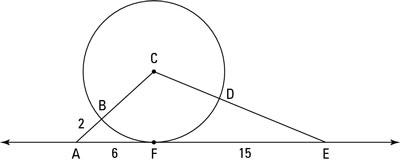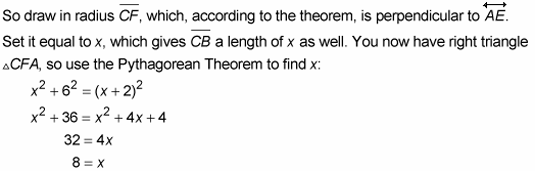##### Geometry For DummiesA line is tangent to a circle if it touches it at one and only one point. If a line is tangent to a circle, then it is perpendicular to the radius drawn to the point of tangency. Check out the bicycle wheels in the below figure.In this figure, the wheels are, of course, circles, the spokes are radii, and the ground is a tangent line. The point where each wheel touches the ground is a point of tangency. And the most important thing — what the theorem tells you — is that the radius that goes to the point of tangency is perpendicular to the tangent line.

Don’t neglect to check circle problems for tangent lines and the right angles that occur at points of tangency. You may have to draw in one or more radii to points of tangency to create the right angles. The right angles often become parts of right triangles (or sometimes rectangles).

Here’s an example problem: Find the radius of circle C and the length of segment DE in the following figure.So the radius is 8. Then you can see that triangle CFE is an 8-15-17 triangle, so CE is 17. (Of course, you can also get CE with the Pythagorean Theorem.) CD is 8 (and it’s the third radius in this problem; does radii, radii, radii ring a bell?). Therefore, DE is 17 – 8, or 9. That does it.